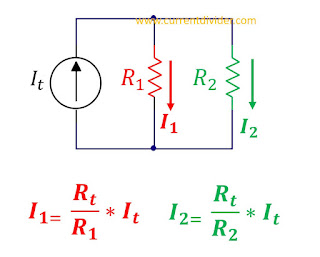# Current Divider

Learn everything about Current Division in Parallel Circuits.

Current divider calculator is a useful tool to calculate the current divided across two resistors. Enter the input current It, and values of two resistors. The two currents will appear.

 It A mA MΩ µA R1 Ω kΩ MΩ GΩ R2 Ω kΩ MΩ GΩ I1 A mA MΩ µA I2 A mA MΩ µA Format # #.# #.## #.### #.#### #.###### #.##...10 #.###....20### Instructions

1. Enter the overall current of the source.
2. Enter the value of the first resistor.
3. Enter the value of the second resistor.
4. The default prefix is in ohms, However, you can change it.
5. By default, the output current is accurate up to 2 digits.
In action:
The screenshot below displays the application of current divider rule to the same circuit which was previously calculated by the manual method.